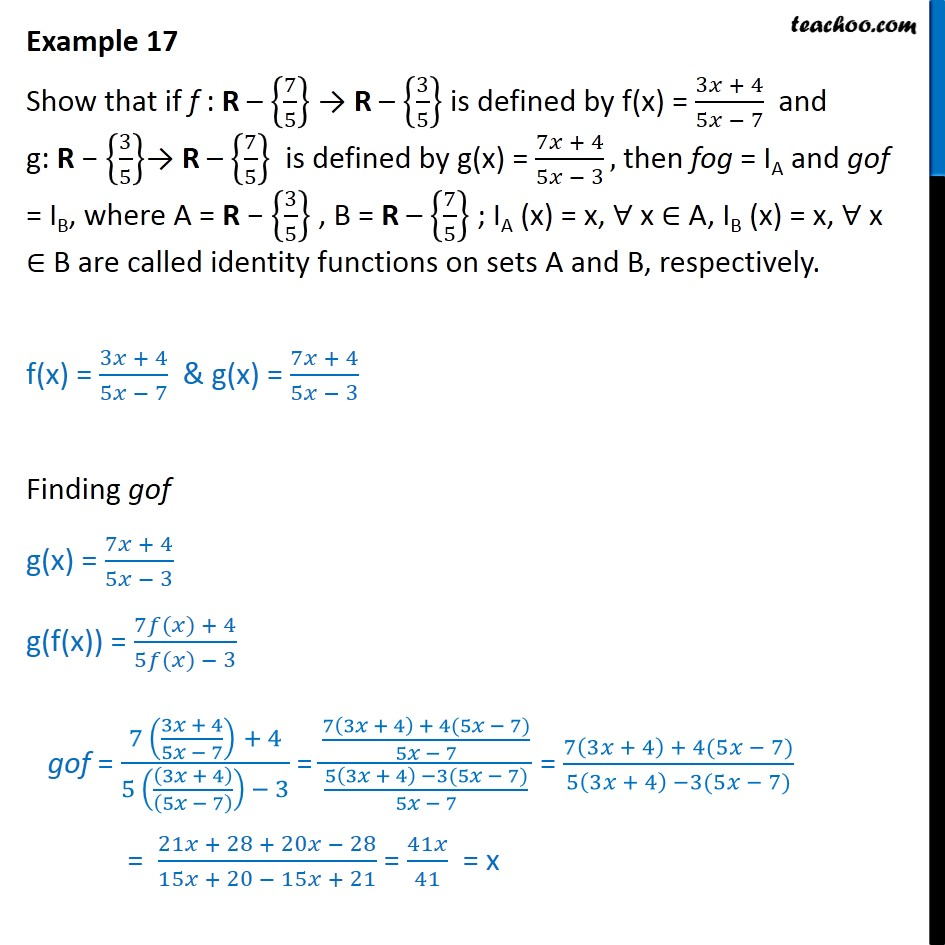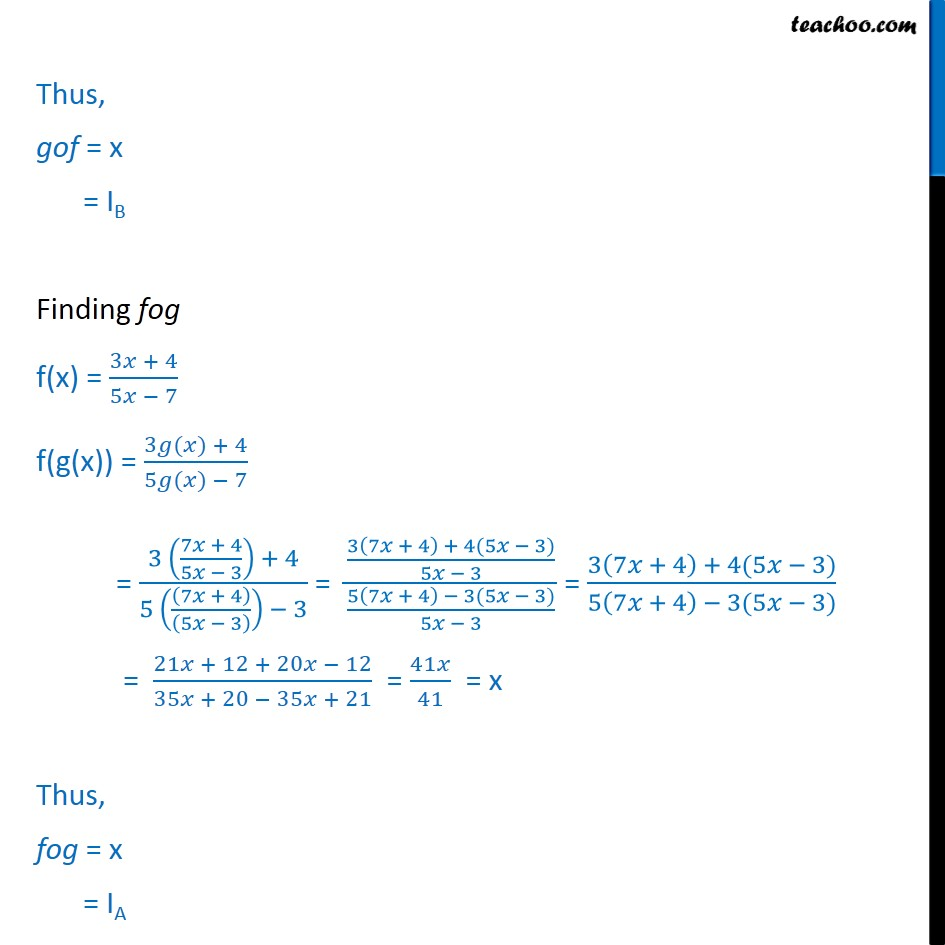1. Chapter 1 Class 12 Relation and Functions
2. Concept wise
3. Composite functions

Transcript

Example 17 Show that if f : R 7 5 R 3 5 is defined by f(x) = 3 + 4 5 7 and g: R 3 5 R 7 5 is defined by g(x) = 7 + 4 5 3 , then fog = IA and gof = IB, where A = R 3 5 , B = R 7 5 ; IA (x) = x, x A, IB (x) = x, x B are called identity functions on sets A and B, respectively. f(x) = 3 + 4 5 7 & g(x) = 7 + 4 5 3 Finding gof g(x) = 7 + 4 5 3 g(f(x)) = 7 ( ) + 4 5 ( ) 3 gof = 7 3 + 4 5 7 + 4 5 3 + 4 5 7 3 = 7 3 + 4 + 4(5 7) 5 7 5 3 + 4 3(5 7) 5 7 = 7 3 + 4 + 4(5 7) 5 3 + 4 3(5 7) = 21 + 28 + 20 28 15 + 20 15 + 21 = 41 41 = x Thus, gof = x = IB Finding fog f(x) = 3 + 4 5 7 f(g(x)) = 3 ( ) + 4 5 ( ) 7 = 3 7 + 4 5 3 + 4 5 7 + 4 5 3 3 = 3 7 + 4 + 4(5 3) 5 3 5 7 + 4 3(5 3) 5 3 = 3 7 + 4 + 4(5 3) 5 7 + 4 3(5 3) = 21 + 12 + 20 12 35 + 20 35 + 21 = 41 41 = x Thus, fog = x = IA

Composite functions